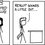# A fun problem - find the formula of number of functions from a power set to another set.

Hi people! This is yet another problem from the entrance test to CMI (Chennai Mathematical Institute) (http://www.cmi.ac.in/).

Consider sets $\displaystyle A = \{1,2,...,k\}$ and $\displaystyle B = \{1,2,...,n\}$. Denote $P_k$ as the power set of $A$. How many functions $f$ can be defined from $P_k$ to $B$ such that $f( M \cup N ) = \text{max} ( f(M), f(N) )$?

Example: For $k = 2$, this function is valid:

1. $f( \phi ) = 2$

2. $f(\{1\}) = 3$

3. $f(\{2\}) = 5$

4. $f(\{1\} \cup \{2\} ) = f( \{1, 2 \} ) = \text{max} ( f(\{1\}), f(\{2\}) ) = 5$

While the following function is invalid:

1. $f( \phi ) = 2$

2. $f(\{1\}) = 3$

3. $f(\{2\}) = 5$

4. $f(\{1\} \cup \{2\} ) = f( \{1, 2 \} ) = 3$

Your answer must be a formula involving $n, k$ only. For $n = 4, k = 3$, the number of such functions is $100$.

I had fun solving it!Note by Parth Thakkar
6 years, 11 months ago

This discussion board is a place to discuss our Daily Challenges and the math and science related to those challenges. Explanations are more than just a solution — they should explain the steps and thinking strategies that you used to obtain the solution. Comments should further the discussion of math and science.

When posting on Brilliant:

• Use the emojis to react to an explanation, whether you're congratulating a job well done , or just really confused .
• Ask specific questions about the challenge or the steps in somebody's explanation. Well-posed questions can add a lot to the discussion, but posting "I don't understand!" doesn't help anyone.
• Try to contribute something new to the discussion, whether it is an extension, generalization or other idea related to the challenge.
• Stay on topic — we're all here to learn more about math and science, not to hear about your favorite get-rich-quick scheme or current world events.

MarkdownAppears as
*italics* or _italics_ italics
**bold** or __bold__ bold
- bulleted- list
• bulleted
• list
1. numbered2. list
1. numbered
2. list
Note: you must add a full line of space before and after lists for them to show up correctly
paragraph 1paragraph 2

paragraph 1

paragraph 2

[example link](https://brilliant.org)example link
> This is a quote
This is a quote
    # I indented these lines
# 4 spaces, and now they show
# up as a code block.

print "hello world"
# I indented these lines
# 4 spaces, and now they show
# up as a code block.

print "hello world"
MathAppears as
Remember to wrap math in $$ ... $$ or $ ... $ to ensure proper formatting.
2 \times 3 $2 \times 3$
2^{34} $2^{34}$
a_{i-1} $a_{i-1}$
\frac{2}{3} $\frac{2}{3}$
\sqrt{2} $\sqrt{2}$
\sum_{i=1}^3 $\sum_{i=1}^3$
\sin \theta $\sin \theta$
\boxed{123} $\boxed{123}$

## Comments

Sort by:

Top Newest

Is it$\displaystyle \sum_{i=1}^n i^k$?

- 6 years, 11 months ago

Log in to reply

That it is! Great!

- 6 years, 11 months ago

Log in to reply

Other problems I found interesting:

- 6 years, 11 months ago

Log in to reply

×

Problem Loading...

Note Loading...

Set Loading...